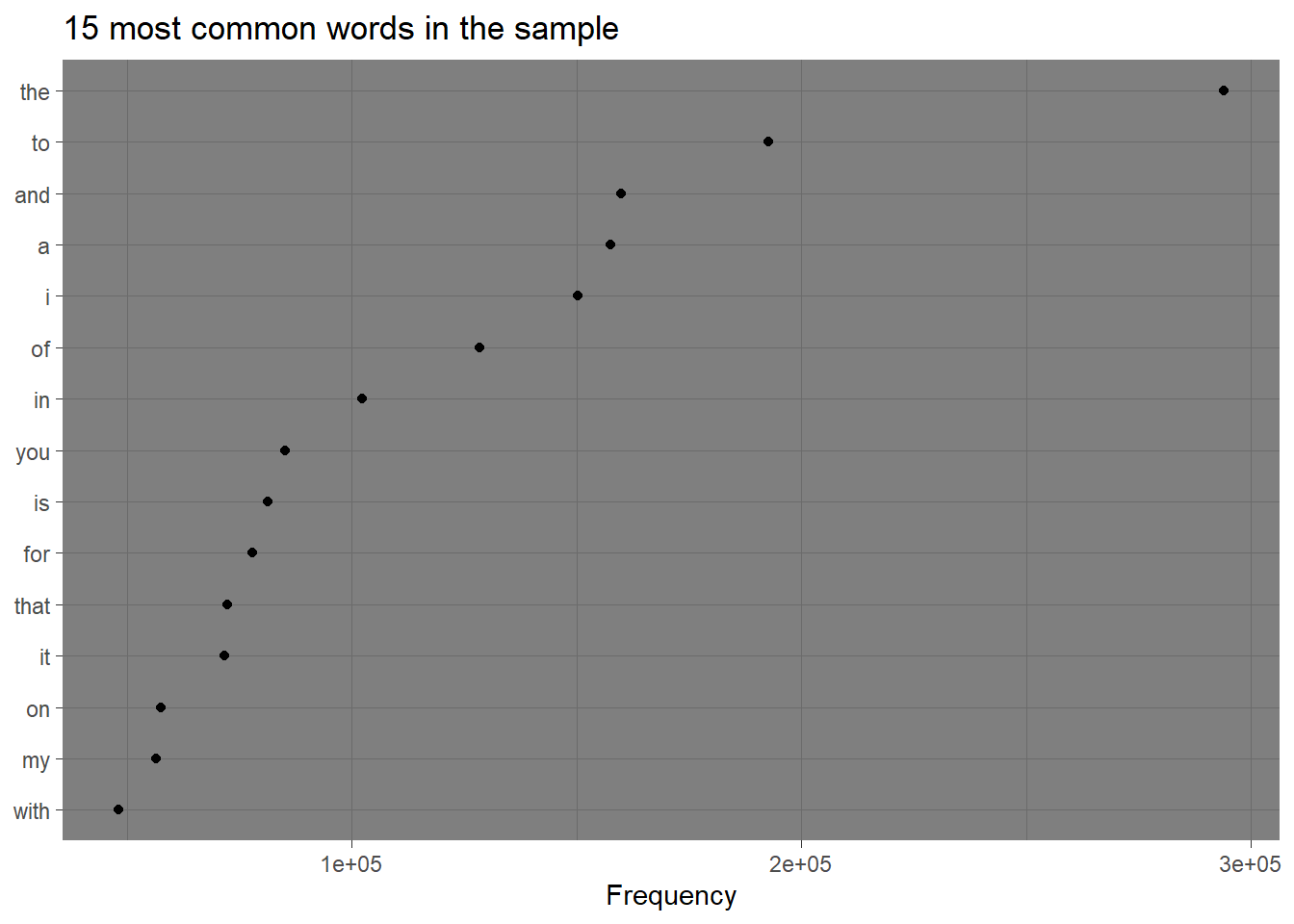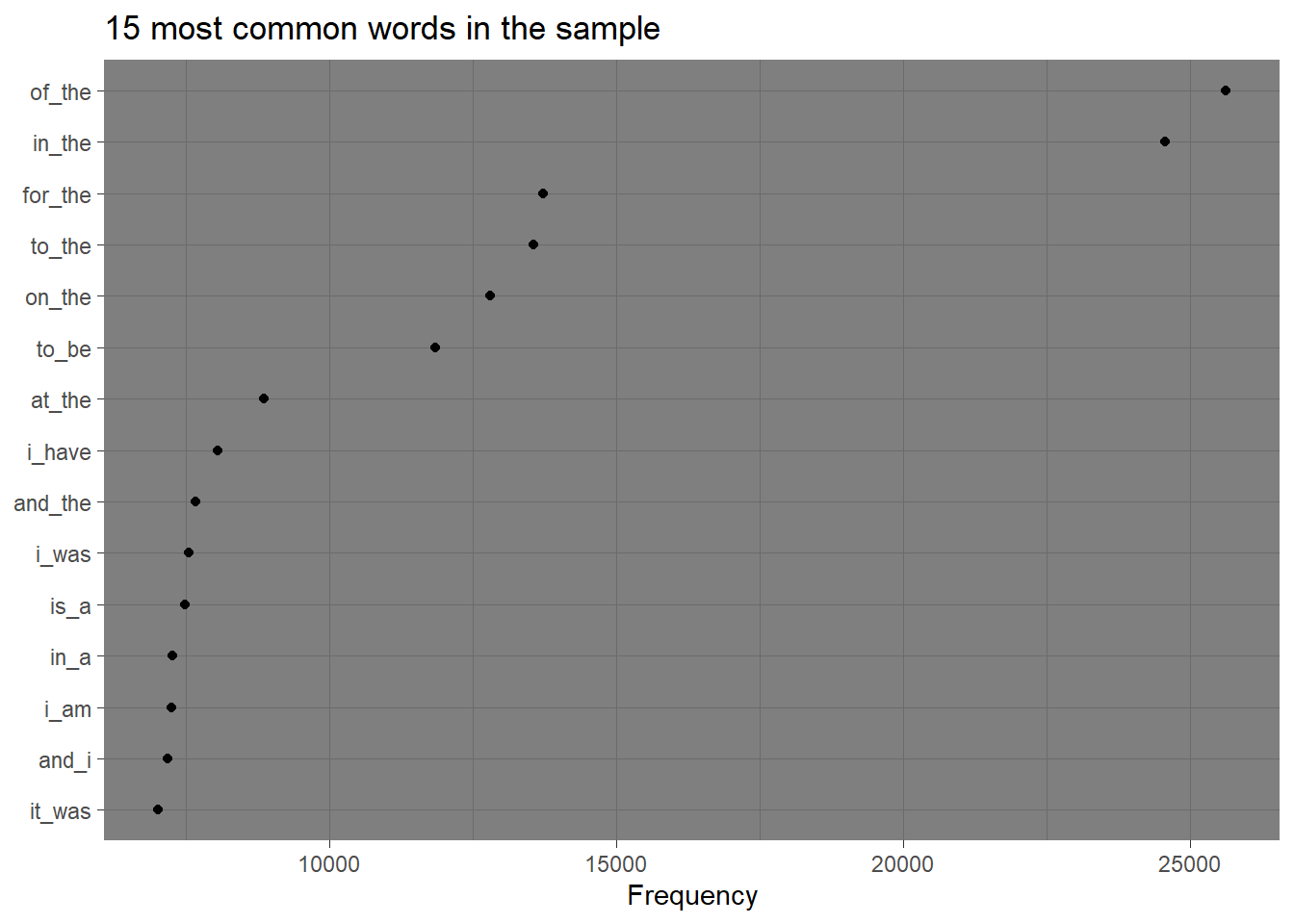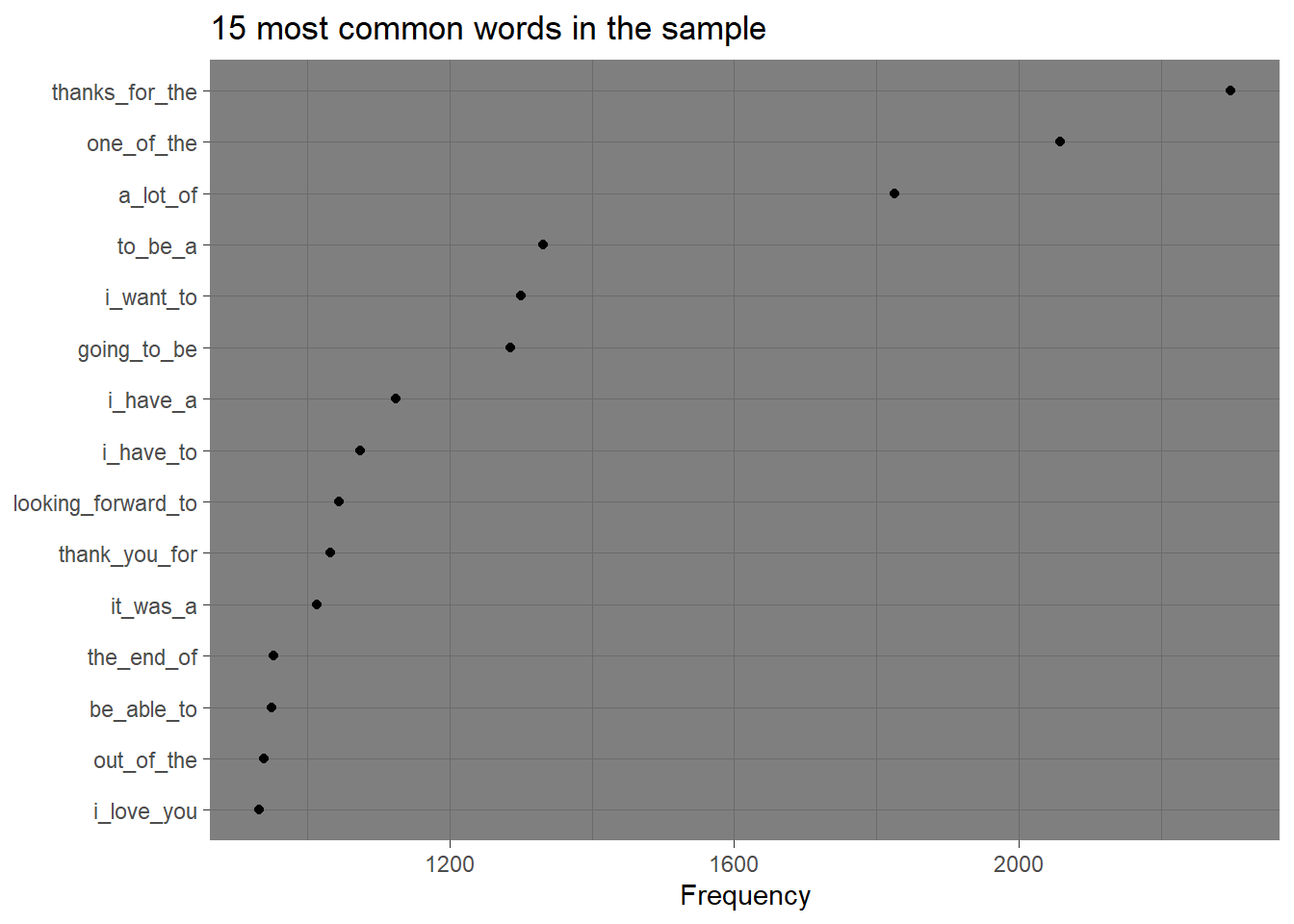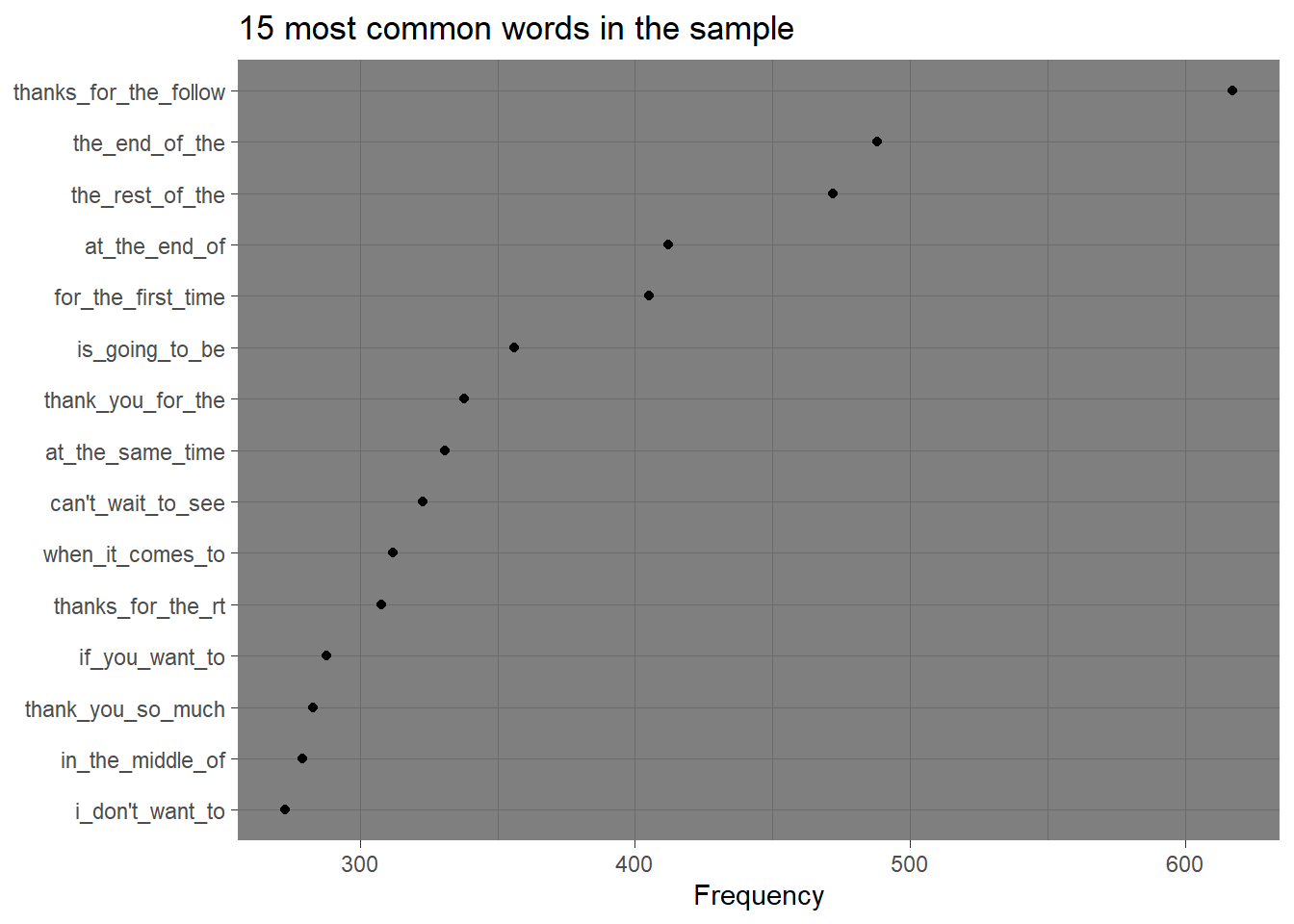if(!file.exists("data.zip"))
{
url = "https://d396qusza40orc.cloudfront.net/dsscapstone/dataset/Coursera-SwiftKey.zip"
unzip("data.zip")
}

## Importing required libraries

suppressMessages(library(quanteda))
suppressMessages(library(stringr))
suppressMessages(library(ngram))
suppressMessages(library(ggplot2))

blogs <- readtext(file = "final/en_US/en_US.blogs.txt", encoding = "UTF-8")

news <- readtext(file = "final/en_US/en_US.news.txt", encoding = "UTF-8")

tweets <- readtext(file = "final/en_US/en_US.twitter.txt", encoding = "UTF-8")

## Summaries of three files

summaries <- data.frame(filename = c('Blogs','News','Tweets'), line.count = c((str_count(blogs$text, "\n") + 1),(str_count(news$text, "\n") + 1),(str_count(tweets$text, "\n") + 1)), word.count = c(wordcount(blogs$text),wordcount(news$text),wordcount(tweets$text)), character.count = c(nchar(blogs$text),nchar(news$text),nchar(tweets\$text)) )

print(summaries)
##   filename line.count word.count character.count
## 1    Blogs     899288   36434844       207723792
## 2     News      77259    2566711        15716666
## 3   Tweets    2360148   28013398       164456178

## Sampling data

5% of all the sentences from the three files will be considered to build the model. This will help in reasonable consumption of memory and time in order to compute.

blogs_sent_crp <- corpus(blogs) %>% corpus_reshape(to = "sentences")
news_sent_crp <- corpus(news) %>% corpus_reshape(to = "sentences")
tweets_sent_crp <- corpus(tweets) %>% corpus_reshape(to = "sentences")
all_corpus <- c(blogs_sent_crp,news_sent_crp,tweets_sent_crp)

rm(blogs_sent_crp)
rm(news_sent_crp)
rm(tweets_sent_crp)
set.seed(5)
sampled_corpus <- sample(all_corpus,size = length(all_corpus)*0.05,replace = FALSE)

## Tokenization and building 2,3 and 4 grams from tokens

all_tokens <- tokens(sampled_corpus,remove_punct = TRUE,remove_symbols = TRUE, remove_numbers = TRUE)

rm(all_corpus)
rm(sampled_corpus)

two_grams <- tokens_ngrams(all_tokens,n=2)
three_grams <- tokens_ngrams(all_tokens,n=3)
four_grams <- tokens_ngrams(all_tokens,n=4)

## Analysis of 1-grams

dfm_obj <- dfm(all_tokens)

textstat_frequency(dfm_obj,n = 15) %>%
ggplot(aes(x = reorder(feature, frequency), y = frequency)) +
geom_point() +
coord_flip() +
labs(x = NULL, y = "Frequency") +
theme_dark() +
ggtitle("15 most common words in the sample")words <- textstat_frequency(dfm_obj) %>% nrow()

Total unique words in the sample: 179185

## Analysis of 2-grams

dfm_obj <- dfm(two_grams)

textstat_frequency(dfm_obj,n = 15) %>%
ggplot(aes(x = reorder(feature, frequency), y = frequency)) +
geom_point() +
coord_flip() +
labs(x = NULL, y = "Frequency") +
theme_dark() +
ggtitle("15 most common words in the sample")words <- textstat_frequency(dfm_obj) %>% nrow()

Total unique pair of words in the sample: 1880559

## Analysis of 3-grams

dfm_obj <- dfm(three_grams)

textstat_frequency(dfm_obj,n = 15) %>%
ggplot(aes(x = reorder(feature, frequency), y = frequency)) +
geom_point() +
coord_flip() +
labs(x = NULL, y = "Frequency") +
theme_dark() +
ggtitle("15 most common words in the sample")words <- textstat_frequency(dfm_obj) %>% nrow()

Total unique triplets in the sample: 4104395

## Analysis of 4-grams

dfm_obj <- dfm(four_grams)

textstat_frequency(dfm_obj,n = 15) %>%
ggplot(aes(x = reorder(feature, frequency), y = frequency)) +
geom_point() +
coord_flip() +
labs(x = NULL, y = "Frequency") +
theme_dark() +
ggtitle("15 most common words in the sample")words <- textstat_frequency(dfm_obj) %>% nrow()

Total unique quadruples in the sample: 5021540

## Plans for prediction algorithm and Shiny application

The next step will be to use Katz’s back-off model for determining the word that should most likely (word with highest probability) appear after a sequence of words. The plan later will be to build a Shiny application where the user will type in words and the system will predict the next word using the algorithm mentioned above.